#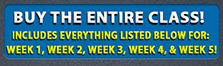Or you may purchase tutorials by the Week below.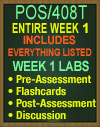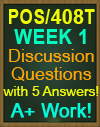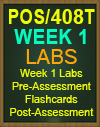# POS/408T Week 1

INCLUDES EVERYTHING LISTED FOR WEEK 1! A+ Work!

POS/408T Week 1 Labs Assignment (with Answers)! A+ Work!

• LAB 1: Finding the largest number
• LAB 2: Understanding one-dimensional array
• LAB 3: Initializing an array and using linear search
• LAB 4: Comparing strings
• LAB 5: Extracting a substring from a string
• LAB 6: Concatenating strings
• LAB 7: Converting a string to lower case

When you complete the lab, be sure to select Submit >> Evaluate to view your lab results. Click Record my answer to save and record your answer to your course.

WK1 Post-Assessment (NEW)

NOTE: Questions are probably not in the same order, Please check below to make sure your questions are on the quiz.

1. What is a function of the Solution Explorer window in Visual Studio?
2. Which of the following statements are true for the above code?
3. What will be the output of the following code?
4. Which of the following applications provide services for supporting a broad variety of clients, including mobile devices and browsers?
5. What will be the output of the following code?
6. Which of the following contains APIs for basic actions that most developers need a program to do?
7. What will be the output of the following C# program?
8. Which of the following is responsible for the execution of all applications developed using the .NET library?
9. What is the error in the following C# program?
10. Which of the following is an application that has the look and feel of a standard Windows application?

WK1 Pre-Assessment

40 Questions & Answers! A+ Work!
NOTE: Questions are probably not in the same order, Please check below to make sure your questions are on the quiz.

1. Which of the following specifies the space to leave blank around the edges of controls?
2. Which of the following statements is correct regarding the given C# program?
3. What will be the output of the following C# program?
4. Which of the following statements are true about partial methods? Each correct answer represents a complete solution. Choose all that apply.
5. Consider the following code. What will be the output if we remove the second partial class definition from the given code?
6. Which of the following is not true about exception handling in C#?
7. Which of the following statements is true about a ComboBox?
8. What will be the output of the given code?
9. Consider the following code. At which line should you place the breakpoint to enter break mode just before the error is caused on the 5,000th cycle?
10. Which of the following statements is true in respect to the following code?
11. Consider the following code. Which of the following is true regarding the given code?
12. How many number of constructors can be defined in a class?
13. What will be the output of the following code?
14. Which of the following are instructions that can interrupt application execution with a user‐defined message?
15. Consider the following code snippet showing object hierarchy. If you instantiate MyDerivedClass using the MyDerivedClass myObj = new MyDerivedClass(); statement, then what will be the sequence of events that occur?
16. Which of the following properties allows a user to edit the text in the TextBox when this is set to true?
17. What will be the output of the following code?
18. What will be the output of the following code?
19. Why is PCL taken into consideration? Each correct answer represents a complete solution. Choose all that apply.
20. Which of the following windows shows the way in which the program reached the current location?
21. Consider the following C# program.
22. Each correct answer represents a complete solution. Choose all that apply.
23. What is the output of the following C# program?
24. What will be the output of the following code?
25. Which of the following control events occurs continually as the mouse travels over the control?
26. What kind of information is transferred to the WPF when a class implements the INotifyPropertyChanged interface?
27. Which of the following binding components specifies the object on which binding is used?
28. What will be the value of the Boolean variable result if a = true and b = false for the following expression?
29. Which of the following control program flow options runs to the end of the code block and resumes the break mode at the statement that follows?
30. Consider the following object hierarchy. If you instantiate MyDerivedClass using the MyDerivedClass myObj = new MyDerivedClass(); statement, then what will be the order of events that will occur?
31. Which of the following panels enables flexible positioning of child controls?
32. Which of the following is the correct way to declare a delegate for calling the function f() defined in the given program?
33. Consider the following code. Which line of code will you use to instantiate MyNestedClass from outside MyClass?
34. Which of the following is the correct order of the given operators in C#?
35. Which layout control holds a single child that will be sized to completely fill this control?
36. Which of the following is the correct statement about an exception?
37. What is the use of the Immediate window in Visual Studio?
38. Which of the following is the correct way of implementing an interface addition by the class maths?
39. Which of the following statements is true for the given lines of code?
40. How a developer is able to display a message on the output window without a temporary pause at the point of debugging?

WK1 Flashcards
Study the flashcards to ensure you understand this week's lesson. Note: Use the Glossary of terms to review terms and definitions.

NOTE: Discussion Question may be different, depending on which Instructor you get. Thanks!

WK1Discussion Question – Runtime Environments

Includes 6 FULL Answers to Discussion Question! A+ Work!

Discuss the .NET runtime environment and the Java® runtime environment. How does this help you better understand the .NET runtime?
Discuss the differences among the .NET environment, Visual Studio®, and the C# programming language. In your discussion, identify and discuss the different components of a Visual Studio C# solution, including files generated by the IDE.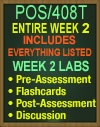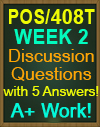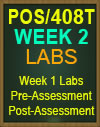POS/408T Week 2

Everything listed for Week 2 is included in purchase!!

Week 2 Labs Assignment (with Answers)! A+ Work!

Week 2 Lab(s) Assignment Instructions
Using the link immediately following these instructions, complete the following labs:

• LAB 1: Using the if-else double-selection statement
• LAB 2: Using the switch statement
• LAB 3: Using the while loop
• LAB 4: Understanding the do-while loop
• LAB 5: Using the for loop-Part 1
• LAB 6: Using the for loop-Part 2
• LAB 7: Understanding functions-Part 1
• LAB 8: Understanding functions-Part 2

WK2 Pre & Post-Assessment

NOTE: Questions are probably not in the same order, Please check below to make sure your questions are on the quiz.

WK2 Flashcards
Study the flashcards to ensure you understand this week's lesson. Note: Use the Glossary of terms to review terms and definitions.

POS/408 WEEK 2 Discussion Question - Creating a C# Program

Includes 10 Responses/Answers to Discussion Question! A+ Work!

Review this week's reading "Unicode and .NET" on the C# in Depth website on the storage requirements for different C# data types. Identify the minimum and maximum value for each type.
Discuss the role of C# data types and variable in C# programming. How does using Unicode or non-Unicode data type impact storage of data on disk or in memory? What do you see as the advantages and disadvantages for each
Create a program that demonstrates the following different types of C# variable scope: Class-Level, Method-Level, and Nested. Post your code in the discussion forum.
Discuss another example from a situation you might face in which you would use one of the types of scope.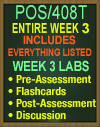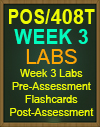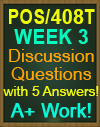POS/408T Week 3

Everything listed for Week 3 is included in purchase!!

Week 3 Labs Assignment (with Answers)! A+ Work!

Week 3 Lab(s) Assignment Instructions
Using the link immediately following these instructions, complete the following labs:

• LAB 1: Learning the using statement
• LAB 2: Handling an Exception
• LAB 3: Using the finally Block
• LAB 4: Converting a string to upper case
• LAB 5: Using the replace function
• LAB 6: Using the trim function

When you complete the lab, be sure to select Submit >> Evaluate to view your lab results. Click Record my answer to save and record your answer to your course.

WK3 Pre & Post-Assessment

NOTE: Questions are probably not in the same order, Please check below to make sure your questions are on the quiz.

WK3 Flashcards
Study the flashcards to ensure you understand this week's lesson. Note: Use the Glossary of terms to review terms and definitions

Week 3 Discussion - Understanding 'if' Statements and Recursion

Includes 10 Full Answers! A+ Work!

Apply conditional statements in C# programming by providing an example of a nested if statement using various logical operators. Include else statements and at least one negation operator.
Discuss different ways to code the following:

• If an account balance is less than \$100, warn the account holder according to the following rules:
• if the balance is between \$50 and \$100 warn the account holder that the balance is low
• if the balance is between \$25 and \$50 then warn the account holder that their balance is critically low
• if the balance is less than \$25 warn the account holder to call the bank.

Recursion is a different way to implement repeating statements. Some problems can only be solved with recursion.
Implement an example of recursion in C#.
Discuss problems with recursion. In what situations must recursion be used?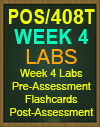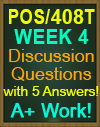POS/408T Week 4

Everything listed for Week 4 is included in purchase!!

Week 4 Labs Assignment (with Correct Answers)! A+ Work!

Week 4 Lab(s) Assignment Instructions
Using the link immediately following these instructions, complete the following labs:

• LAB: Using access modifiers
• LAB: Using the switch statement with the string
• LAB: Obtaining the sum of even numbers using the for loop
• LAB: Using Delegate Keyword
• LAB: Understanding the variable scope
• LAB: Understanding the operator precedence

When you complete the lab, be sure to select Submit >> Evaluate to view your lab results. Click Record my answer to save and record your answer to your course.

WK4 Pre & Post-Assessment

NOTE: Questions are probably not in the same order, Please check below to make sure your questions are on the quiz.

WK4 Flashcards
Study the flashcards to ensure you understand this week's lesson. Note: Use the Glossary of terms to review terms and definitions.

POS/408T WEEK 4 Discussion Question

Custom Exceptions and Object-Oriented Programming

Includes 20 Full Answers! A+ Work!

Respond to the following in a minimum of 175 words:
The exception class represents errors that occur during application execution.
Create a custom exception class.
Discuss how you would handle the custom exception you created. What do you see as the advantages and disadvantages of custom exceptions?
Demonstrate through code and explanation each of the following object oriented concepts:

• Inheritance
• Encapsulation
• Polymorphism

Discuss the advantages of applying object orienting coding principles.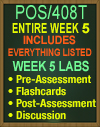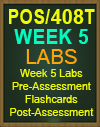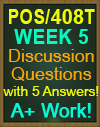POS/408T Week 5

Everything listed for Week 5 is included in purchase!!

Week 5 Labs Assignment (with Answers)! A+ Work!

• LAB: Using the while loop for printing Fionacci series
• LAB: Creating multiple arguments in a function
• LAB: Using the nested for loop for printing a pattern
• LAB: Using the do-while loop
• LAB: Using the if-else statement within the while loop
Pre-Assessment
NOTE: Questions are probably not in the same order, Please check below to make sure your questions are on the quiz.

1. Which of the following control events occurs continually as the mouse travels over the control?
2. What are the features of dependency properties?
3. Which of the following properties allows a user to edit the text in the TextBox when this is set to true?
4. Which of the following specifies the space to leave blank around the edges of controls?
5. Which of the following statements is true about a ComboBox?
6. What kind of information is transferred to the WPF when a class implements the INotifyPropertyChanged interface?
7. Which of the following panels enables flexible positioning of child controls?
8. Which of the following binding components specifies the object on which binding is used?
9. Why is PCL taken into consideration?
10. Which layout control holds a single child that will be sized to completely fill this control? This task contains the radio buttons and checkboxes for options. The shortcut keys to perform this task are A to H and alt+1 to alt+9.

WK5 Flashcards
Study the flashcards to ensure you understand this week's lesson. Note: Use the Glossary of terms to review terms and definitions

WK5 uCertify (NEW) Final Exam

1. Which of the following capabilities are designed using XAML?
2. Which of the following statements are true of a namespace in C# .NET?
3. Which of the following lines of code will be placed on Line 1 to print the required output?
4. Which of the following lines of code is required to compile the given code successfully?
5. Which common layout panel enables flexible positioning of child controls?
6. In which of the following situations can a code enter in the break mode?
7. What will be the value of ob2.a after line 1 in the following lines of code?
8. What do you understand by managed codes?
9. Which of the following expressions is the odd one out?
10. Consider the given C# program. Which of the following code snippets are appropriate to complete the given code for producing the required output?
11. What will be the output of the following C# program?
12. In which of the following situations can a hidden member access the base class member from the derived class?
13. What will be the output of the following C# program?
14. Which of the following statements are true regarding the following code snippet?
15. Which of the following is an ideal framework for building RESTful HTTP services that support a broad variety of clients?
16. WPF provides a far more flexible and powerful way to create desktop applications by replacing which of the following?
17. Why are assemblies useful while deploying applications?
18. If members are accessible only from code that is part of either the class or a derived class, then which accessibility level should be used?
19. What will be the output of the following code?
20. What is the output of the following C# program?
21. What will be the output of the following C# program?
22. Which of the following are the bitwise operators in C#?
23. Considering the following code snippet, which of the following statements are correct?
24. Which of the following statements is true regarding the given C# program?
25. What is the role of a solution in Visual Studio?
26. What are the correct ways to initialize the variables x and y to a value 5 each?
27. What will be the output of the following C# program?
28. In which of the following situations can a code enter in the break mode?
29. What will be the output of the following C# program?
30. Which Visual Studio tool will you use to find and use all available objects of a project and view their properties, methods, and events?

1. Consider the given C# program (“Mango”, “Apple”, “Cherry”). Which of the following code snippets are appropriate to complete the given code for producing the required output?
2. Which of the following Code snippets are appropriate to complete the given code for producing the require output?
3. In which of the following situations can a code enter in the break mode?
4. What will be the output of the following C# program?
5. What will be the output of the following C# program?
6. Consider the following C# program. Which of the following lines of code will be placed on Line 1 to print the required output?
7. What will be the output of the following C# program?

Post-Assessment
NOTE: Questions are probably not in the same order, Please check below to make sure your questions are on the quiz.

1. Which of the following statements is true for the given lines of code? If you instantiate myDerivedClass using the MyDerivedClass myObj = new MyDerivedClass(); statement, then what will be the sequence of events that occur?
2. Which of the following statements is true for the given lines of code?
3. Which of the following statements is true in respect to the following code?
4. What will be the output of the following code?
5. Which of the following is the correct way to declare a delegate for calling the function f() defined in the given program?
6. Which of the statements is correct regarding the given C# program?
7. What is the output of the following C# program?
8. Which of the following is the correct statement about an exception?
9. What will be the output of the following code?
10. What will be the output of the following code?
11. What is the use of the immediate window in Visual Studio?
12. Which of the following is true regarding the given code?
13. What will be the value of the Boolean variable result if a = true and b = false for the following expression?
14. Which of the following control program flow options runs to the end of the code block and resumes the break mode at the statement that follows?
15. What will be the output of the following code?
16. Which of the following is responsible for the execution of all applications developed using the .NET Library?
17. How many number constructors can be defined in a class?
18. Which of the following is an application that has the look and feel of a standard Windows application?
19. Consider the following code.    Which line of code will you use to instantiate MyNestedClass from the outside MyClass?
20. Consider the following code. At which line should you place the breakpoint to enter break mode just before the error is caused on the 5,000th cycle?
21. Consider the following code. Which of the following statements are true for the above code? Each correct answer represents a complete solution. Choose all that apply.
22. What will be the output of the following code?
23. Which of the following instructions that can interrupt application execution with a user-defined message?
24. Which of the following windows shows the way in which the program reached the current location?
25. What will be the output of the following C# program?
26. Consider the following C# program. Which of the following return functions m() print the output as 3?
27. What will be the output of the following C# program?
28. Which of the following is not true about exception handling in C#?
29. What will be the output of the given code?
30. Consider the following code. What will be the output if we remove the second partial class definition from the given code?
31. Which of the following contains APIs for basic actions that most developers need a program to do?
32. Which of the following applications provide services for supporting a broad variety of clients, including mobile device and browser?
33. Which of the following statements are true about partial methods?
34. How a developer is able to display a message on the output window without a temporary pause at the point of debugging?
35. What is the error in the following C# program?
36. What will be the output of the following code?
37. Which of the following is the correct order of the given operators in C#?
38. Consider the following object hierarchy.   If you instantiate MyDerivedClass using the MyDerivedClass myObj = new MyDerivedClass(); statement, then what will be the order of events that will occur?
39. Which of the following is the correct way of implementing an interface addition by the class maths?
40. What is a function of the Solution Explorer window in Visual Studio?

Pluralsight Skill IQ C# Assessment

NOTE: Questions are probably not in the same order, Please check below to make sure your questions are on the quiz.

Pluralsight Quiz #1 (NEW)

1. What best describes the SelectMany () Language integrated Query (LINQ) extension method?
2. What type of key is being used in this Language Integrated Query (LINQ) to join the data from three tables?
3. Given these two method overloads:  What will the following code do?
What does the (ServiceContract) attribute do in this interface class?
4. What steps are required to initialize and handle the NumberRandomized event?
5. What will the value of fact2 be after the code executes?
6. Consider this code public static class MyClass { // etc.   What effect does the keyword static have on the class?
7. Regarding polymorphism, which statement is true?
8. What type of key must you use to group by more than one key in a Language Integrated Query (LINQ) query?
9. Review the following snippet of code: A7.B7.Do (C);  In regards to the statement “null-conditional operators are short-circuiting”, which statement is true?
10. Which statement best describes the following class?
11. Where would you normally use the C# await operator?
12. You have three DateTime Value: dateToCheck   beginningDate  endingDate      Assuming that beginningDate is less than endingDate, which line of code will return a Boolean value indicating if the dateToCheck value is within the beginningDate and endingDate range?
13. Which of the following is the correct syntax for declaring and initializing an array of int?
14. The following snippet of code is using LINQ to return the count of the unique values in a Dictionary<TKey, TValue>. The count is returning the number of items in the dictionary and not the unique value count, however var uniqueCount = dictionary.Values.Count();    What must you change to return the correct number of unique values?
15. What is the difference between Action<T> and Func<TResult>?
16. This Employee class implements the interface IEmployee but there is a compiler error. What is the cause of this error?
Alternate Pluralsight Quiz #2

1. Consider the following variable declaration.

var quote = "If we knew what it was we were doing, it would not be called  research-Albert Einstein";. Which choice

will correctly split quote to produce these values?

2. Which method is the safest way to convert an unknown string value to a numeric value?

3. Considering the following snippet of code, where will the break statement cause execution to transfer to?

4. By using interfaces, you can include behavior from multiple sources in a class. Why is this capability especially

important in regards to C#?

5. Which statement best describes the following class?

6. Which statement describes this line of code?

7. Both of the following classes have methods named DoMath(). Both return integer

values and both are within the scope of the code you are writing, but they perform

very different functions. Which code snippet correctly calls the class Math1's DoMath

() method?

8. The following code is not compiling. What is the problem with this block of code?

9. What is the correct process to explicitly declare a variable with the data type of string and set the value to "cat"?"

10. What will the output to the console be after this code executes?

POS/408T Discussion Questions (and 5 Answers)! A+ Work

Wk 5 - Discussion - Applying Data Structures and Sorting

Includes 5 Answers to this DQ! A+ Work!

Knowing when to apply a data structure for a given business problem is an important skill for developers to know.
Research the following .NET data structures:

•   List

•  ArrayList